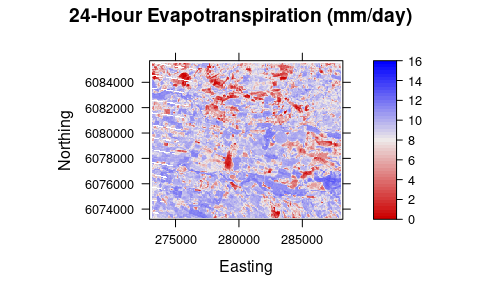# Surface Energy Balance using METRIC model and water package: 1. simple procedure

#### 2018-04-24

This vignette presents the procedure to estimate the Land Surface Energy Balance (LSEB) using lansat imagery and The water package. There are two version of this vignette: simple and advanced version. In the first one the procedure is simpler but most of the parameters are selected automatically by the package. In the second aproach, the procedure is longer because the user has more control on all input parameters and coefficent. Both vignettes follow the METRIC model methodology (Allen et la., 2007) in order to estimate the LSEB using landasat 7 and 8 satellite images.

Also, on the simple procedure we are going to assume flat terrain, but in the advanced procedure we are going to use a digital elevation model (DEM). However, is possible to use a digital elevation model in the simple procedure, changing the parameter flat to FALSE and providing a DEM.

## Introduction

One of the most cited models to estimate land surface evapotranspiration from satellite-based energy balance is the Mapping EvapoTranspiration at high Resolution with Internalized Calibration (METRIC). This model was developed by Allen et al., (2007) based on the well-known SEBAL model (Bastiaanssen, 1998). It has been widely applied in many countries arround the world to estimate crops evapotranspiration (ET) at field scales and over large areas using satellite images. The model it has been apply in different vegetation and crops types such us, wheat, corn, soybean and alfalfa with good results (3 - 20% of error) and also in recent years over sparce woody canopies such us vineyards, and olive orchards, in both plain and mountainous terrain. Thus, ET is estimated as a residual of the surface energy equation: $\begin{equation} \label{eq:EB} LE = R_n - G - H \end{equation}$ where $$LE$$ is latent energy consumed by ET ($$W \cdot m^{-2}$$); $$Rn$$ is net radiation ($$W \cdot m^{-2}$$); $$G$$ is sensible heat flux conducted into the ground ($$W \cdot m^{-2}$$); and $$H$$ = sensible heat flux convected to the air ($$W \cdot m^{-2}$$).

We estimate $$Rn$$, $$G$$ and $$H$$ for each pixel into a Landsat satellite scene, supported by one weather station. Then we estimate $$LE$$ fluxes using previous equation, and after that the instantaneous evapotranspiration values as:

$\begin{equation} ET_{inst} = 3600 \cdot \frac{LE}{\lambda \rho_w} \end{equation}$

where $$ET_{inst}$$ is the instantaneous ET at the satellite flyby ($$mm \cdot h^{-1}$$); 3600 is the convert factor from seconds to hours; $$\rho_w$$ is density of water = 1000 $$kg\cdot m^{-3}$$; and $$\lambda$$ is the water latent heat of vaporization ($$J\cdot kg^{-1}$$).

Finally the daily ET is computed pixel by pixel (30 x 30 m) as: $\begin{equation} ET_{24} = \frac{ET_{inst}}{ET_r} ET_{r\_24} \label{eq:et24} \end{equation}$

To begin this procedure, first load water package:

library(water)

## Base data preparation

To calculate METRIC crops Evapotranspiration using water package, and the simple procedure we’re going to use three sources:

• A raw Landsat 7-8 satellite image (original .TIF data from glovis USGS).
• A Weather Station data (.CSV file).
• A polygon with our Area-of-interest (AOI) Spatial-Polygon object (if we wont estimate corp ET for the entire landsat scene).

First, we create the AOI as a polygon using bottomright and topleft points:

aoi <- createAoi(topleft = c(272955, 6085705),
bottomright = c( 288195, 6073195), EPSG = 32719)

Then, we load the weather station data. For that we are going to use the function called read.WSdata. This function converts our .csv file into a waterWeatherStation object. Then, if we provide a Landsat metadata file (.MTL file) we will be able to calculate the time-specific weather conditions at the time of satellite overpass.

csvfile <- system.file("extdata", "apples.csv", package="water")
MTLfile <- system.file("extdata", "L7.MTL.txt", package="water")
WeatherStation <- read.WSdata(WSdata = csvfile, date.format = "%d/%m/%Y",
lat=-35.42222, long= -71.38639, elev=201, height= 2.2,
columns=c("date" = 1, "time" = 2, "radiation" = 3,
"wind" = 4, "RH" = 6, "temp" = 7, "rain" = 8),
MTL = MTLfile)
## Warning in read.WSdata(WSdata = csvfile, date.format = "%d/%m/%Y", lat =
## -35.42222, : As tz = "", assuming the weather station time zone is America/
## Argentina/Buenos_Aires

We can visualize our weather station data as:

print(WeatherStation)
## Weather Station @ lat: -35.42 long: -71.39 elev: 201
## Summary:
##    radiation           wind              RH              ea
##  Min.   :  0.00   Min.   : 0.000   Min.   :17.39   Min.   :0.7455
##  1st Qu.:  0.00   1st Qu.: 0.205   1st Qu.:40.65   1st Qu.:1.2288
##  Median : 49.82   Median : 1.585   Median :64.83   Median :1.6472
##  Mean   :310.13   Mean   : 3.071   Mean   :60.78   Mean   :1.5156
##  3rd Qu.:719.29   3rd Qu.: 3.655   3rd Qu.:82.34   3rd Qu.:1.7741
##  Max.   :998.29   Max.   :14.460   Max.   :94.04   Max.   :1.9672
##       temp            rain
##  Min.   :14.65   Min.   :0
##  1st Qu.:17.74   1st Qu.:0
##  Median :21.15   Median :0
##  Mean   :22.46   Mean   :0
##  3rd Qu.:27.36   3rd Qu.:0
##  Max.   :32.53   Max.   :0
##
##  Conditions at satellite flyby:
##               datetime radiation wind    RH   ea  temp rain       date
## 47 2013-02-15 11:30:40    752.92  1.1 68.86 1.89 22.59    0 2013-02-15
plot(WeatherStation, hourly=TRUE)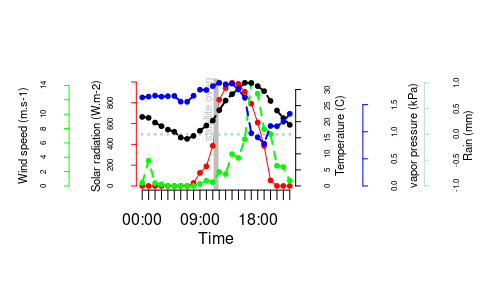After that, we need to load the Landast satellite image. Usually, using water we can use the function called loadImage to load a Landsat image from .TIF files were downloaded directly from Earth Explorer. In this vignette we are going to use some Landsat 7 as example data which, by the way comes with water package as a demo.

image.DN <- L7_Talca
plot(image.DN)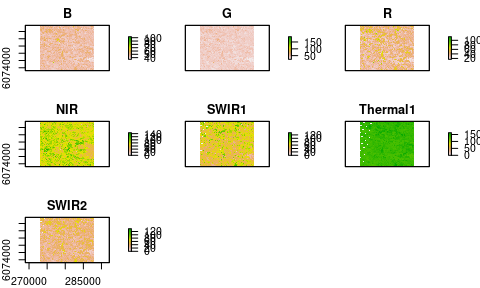## Surface Energy Balance estimation

We are going to use the function called METRIC.EB to estimate the land surface energy balance. This function has many parameters to choose from the different METRIC model equations. e.g., you can change:

• coefficients used to estimate broadband albedo.
• Model to estimate Leaf Area Index (LAI) from satellite data.
• Model to estimate momentum roughness lenght (Zom)
• Automatic method to select anchors pixels
• ETr coefficient and momentum roughness lenght for the weather station

When we run METRIC.EB, the energy balance and the surface temperature (Ts) used are assigned to Energy.Balance object. Also the function prints the position and some other data from the anchors pixels, and finally plots the values of the aerodinamic resistance during the iterative process. Here is a parameter verbose to control how much information we want to see in the output.

Energy.Balance <- METRIC.EB(image.DN = image.DN, plain=TRUE,
WeatherStation = WeatherStation,
ETp.coef = 1.2, MTL=MTLfile, n = 5,
sat="L7", thermalband=image.DN$thermal.low) ## pixel X Y Ts LAI NDVI type ## 1 110205 287250 6079210 327.1150 0.07 0.23 hot ## 2 49291 273390 6082780 307.5172 4.08 0.8 cold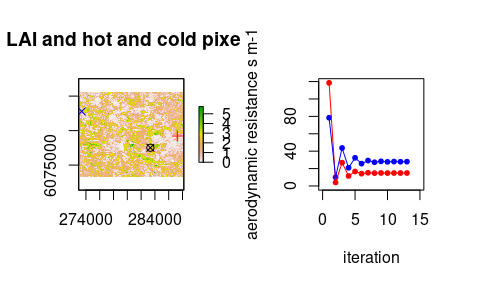Now we can plot the results using: plot(Energy.Balance$EB)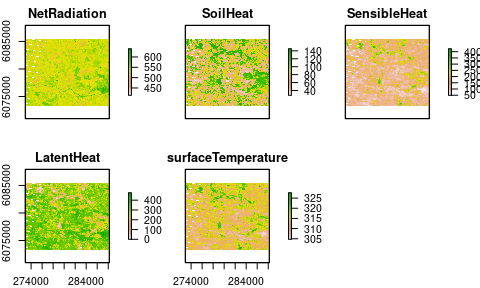## Daily Crop Evapotranspiration (ET_24) estimation

To estimate the daily crop evapotranspiration from the Landsat scene we need the daily reference ET (ETr) for our weather station, so we can calculate the daily ETr with:

ET_WS <- dailyET(WeatherStation = WeatherStation, MTL = MTLfile)

And finally, we can estimate daily crop ET pixel by pixel:

ET.24 <- ET24h(Rn=Energy.Balance$EB$NetRadiation, G=Energy.Balance$EB$SoilHeat,
H=Energy.Balance$EB$SensibleHeat,
Ts=Energy.Balance$EB$surfaceTemperature,
WeatherStation = WeatherStation, ETr.daily=ET_WS)`